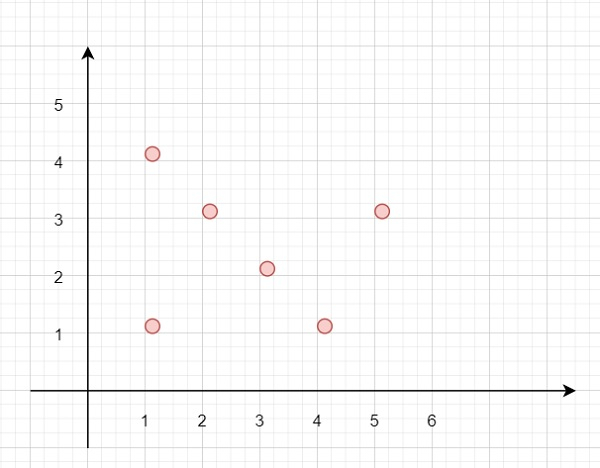# Max Points on a Line in C++

Suppose we have a 2D plane. We have to find the maximum number of points that reside on the same straight line. So if the points are like −Then there are 4 points

To solve this, we will follow these steps −

• n := number of points, if n < 3, then return n

• ans := 2

• for i in range 1 to n – 1

• count := 0

• take two points from index i and i – 1, these are p1, p2

• if p1 and p2 points are same, then

• for j in range 0 to n – 1

• if points[j].x = p1.x and points[j].y = p1.y, then increase count by 1

• otherwise −

• for j in range 0 to n – 1

• p3 := point from index j

• if p3.y – p2.y * p2.x – p1.x = p2.y – p1.y * p3.x – p2.x, then increase count by 1

• ans := max of ans and count

• return ans

## Example

Let us see the following implementation to get a better understanding −

Live Demo

#include <bits/stdc++.h>
using namespace std;
typedef long long int lli;
class Solution {
public:
int maxPoints(vector<vector<int>>& points) {
int n = points.size();
if(n<3)return n;
int ans = 2;
for(int i = 1;i<n;i++){
int count = 0;
lli x1 = points[i-1];
lli x2 = points[i];
lli y1 = points[i-1];
lli y2 = points[i];
if(x1 == x2 && y1 == y2){
for(int j =0;j<n;j++){
if(points[j] ==x1 && points[j] == y1)count++;
}
} else {
for(int j =0;j<n;j++){
int x3 = points[j];
int y3 = points[j];
if((y3-y2)*(x2-x1) == (y2-y1)*(x3-x2))count++ ;
}
}
ans = max(ans, count);
}
return ans;
}
};
main(){
Solution ob;
vector<vector<int>> v = {{1,1},{3,2},{5,3},{4,1},{2,3},{1,4}};
cout << (ob.maxPoints(v));
}

## Input

[{1,1},{3,2},{5,3},{4,1},{2,3},{1,5}]

## Output

4

Updated on: 26-May-2020

305 Views